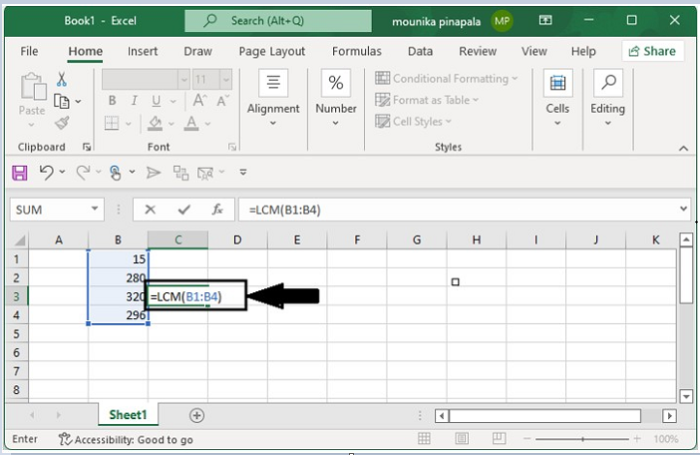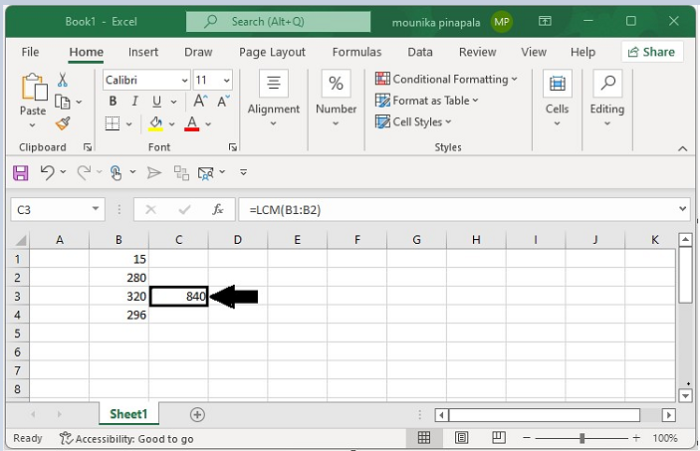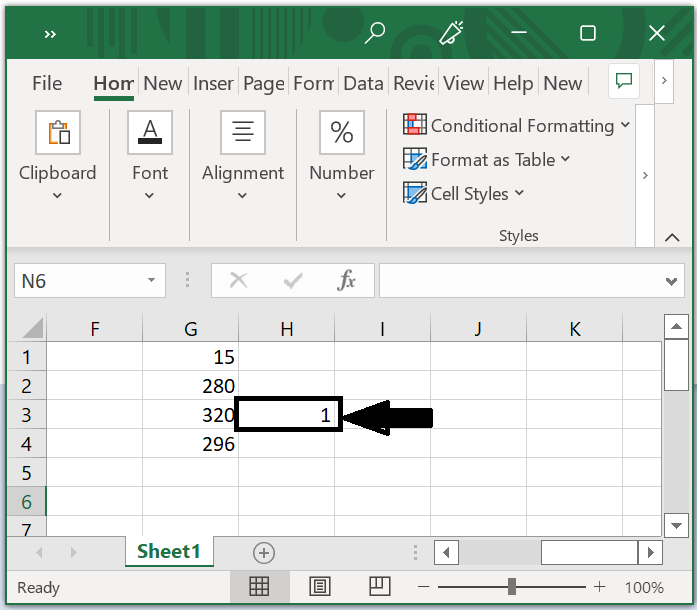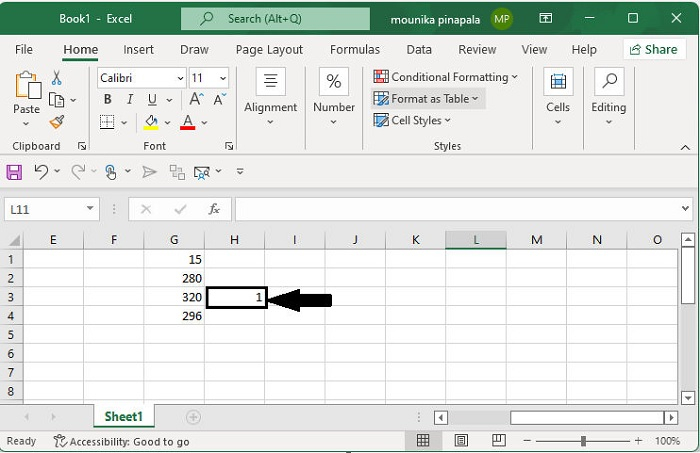# Find the lowest common denominator or greatest common factor in Excel

We have used the lowest common denominator and greatest common factors we are students. However, if there are more big numbers, doing this will be complicated. Greatly Excel provides some functions to get the lowest common denominator or greatest common factor of numbers.

## Finding the Lowest Common Denominator of some numbers in Excel

Lowest Common Multiple

The Lowest Common Multiple or LCM of a set of numbers is the smallest common multiple of those integers. This means all those numbers to be divisible by the LCM giving an integer value. LCM is useful for calculating problems related to gears and planetary alignment.

Kindly apply the LCM function to the given numbers in order to find the lowest common denominator to the given numbers.

Step 1

Open an Excel workbook and enter the numbers in the cells as per your wish. Now enter the formula =LCM(B1:B2) in the blank cell as shown in the below screen shot.Step 2

After pressing the Enter key, you will be able to calculate the least common denominator between all the numbers in the range of cells.## Finding the Greatest Common Factor of Numbers in Excel

Greatest Common Factor/Divisor

The Greatest Common Divisor or GCD is the highest integer number that could be used to divide the set of numbers and gives you an integer value.

Using the GCD function will enable you to calculate the greatest common factor of several numbers if you need to find the common factor between them.

Step 1

Open an Excel workbook and enter the numbers in the cells as per your wish. Now enter the formula =GCD(G1:G4) in the blank cell as shown in the below screen shot.Step 2

After pressing the Enter key, you will be able to calculate Greatest Common factor between all the numbers in the range of cells.Note

• It is important to understand that in the above formulas, B1:B2 indicate the range of cells, of the number that you want to calculate the LCM or GCD.

• Ensure that all values are in the same column, it is important for them to be in the same row.

• The formula would work regardless of whether you miss a cell or two in a column as long as the first and last cells are the same in both columns.